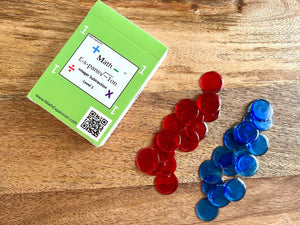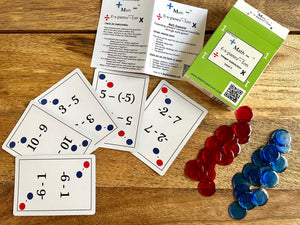# Integer Subtraction Level 1 Card Deck Starter Set

Regular price \$20.00
Unit price  per

Looking for a fun and interactive way to help your scholars excel in math? Look no further! Math Expansion breaks down adding and subtracting positive and negative numbers ("integers") using a hands-on minds-on method. This allows scholars to understand the concept of integers instead of memorizing rules. Scholars are introduced to the concept in Level 1 with numbers -10 to +10. Differentiate your classroom and have loads of fun during family game night while preparing scholars for algebra, college, and beyond!

Integer Subtraction Level 1 introduces scholars to the concept using 1-digit integers. Each deck of Integer Subtraction Level 1 includes the following:

• 1 bag of positive integer counters

• 1 bag of negative integer counters

• A booklet explaining how to use integer counters and how to conduct checks for understanding

• A deck of 52 cards with introductory integer subtraction problems and answers